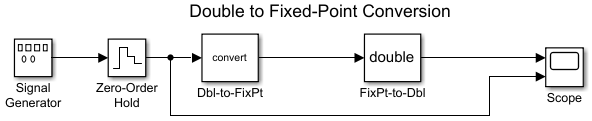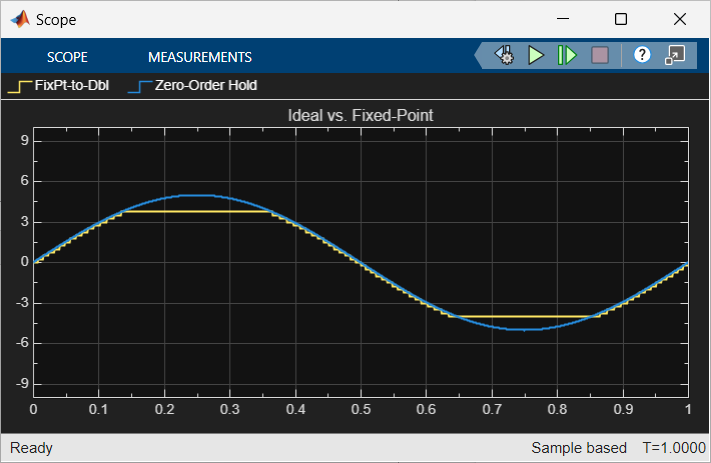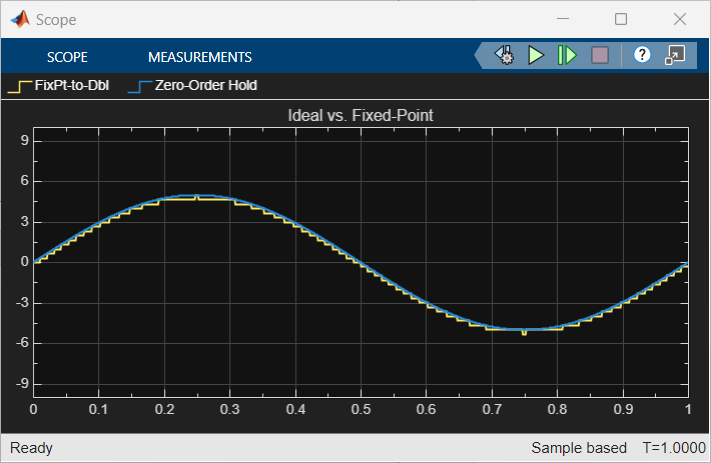## Cast from Doubles to Fixed Point

The purpose of this example is to show you how to simulate a continuous real-world doubles signal using a generalized fixed-point data type. Although simple in design, the model gives you an opportunity to explore many of the important features of the Fixed-Point Designer™ software, including

• Data types

• Scaling

• Rounding

• Logging minimum and maximum simulation values to the workspace

• Overflow handling

This example uses the `fxpdemo_dbl2fix` model. Open the model:

`fxpdemo_dbl2fix`The sections that follow describe the model and its simulation results.

### Block Descriptions

In this example, you configure the Signal Generator block to output a sine wave signal with an amplitude defined on the interval `[-5 5]`. The Signal Generator block always outputs double-precision numbers.

The Data Type Conversion (Dbl-to-FixPt) block converts the double-precision numbers from the Signal Generator block into one of the Fixed-Point Designer data types. For simplicity, the size of the output signal is 5 bits in this example.

The Data Type Conversion (FixPt-to-Dbl) block converts one of the Fixed-Point Designer data types into a Simulink® data type. In this example, it outputs double-precision numbers.

### Simulations

The following sections describe how to simulate the model using binary-point-only scaling and [Slope Bias] scaling.

#### Binary-Point-Only Scaling

When using binary-point-only scaling, your goal is to find the optimal power-of-two exponent E, as defined in Scaling. For this scaling mode, the fractional slope F is 1 and there is no bias.

To run the simulation:

1. Configure the Signal Generator block to output a sine wave signal with an amplitude defined on the interval ```[-5 5]```.

1. Double-click the Signal Generator block to open the `Block Parameters` dialog.

2. Set the Wave form parameter to `sine`.

3. Set the Amplitude parameter to `5`.

4. Click .

2. Configure the Data Type Conversion (Dbl-to-FixPt) block.

1. Double-click the Dbl-to-FixPt block to open the `Block Parameters` dialog.

2. Verify that the Output data type parameter is `fixdt(1,5,2)`. `fixdt(1,5,2)` specifies a 5-bit, signed, fixed-point number with scaling `2^-2`, which puts the binary point two places to the left of the rightmost bit. Hence the maximum value is 011.11 = 3.75, a minimum value of 100.00 = -4.00, and the precision is (1/2)2 = 0.25.

3. Verify that the Integer rounding mode parameter is `Floor`. `Floor` rounds the fixed-point result toward negative infinity.

4. Select the Saturate on integer overflow checkbox to prevent the block from wrapping on overflow.

5. Click .

The Scope displays the real-world and fixed-point simulation results.The simulation shows the quantization effects of fixed-point arithmetic. Using a 5-bit word with a precision of (1/2)2 = 0.25 produces a discretized output that does not span the full range of the input signal.

If you want to span the complete range of the input signal with 5 bits using binary-point-only scaling, then your only option is to sacrifice precision. Hence, the output scaling is `2^-1`, which puts the binary point one place to the left of the rightmost bit. This scaling gives a maximum value of 0111.1 = 7.5, a minimum value of 1000.0 = -8.0, and a precision of (1/2)1 = 0.5.

To simulate using a precision of 0.5, set the Output data type parameter of the Data Type Conversion (Dbl-to-FixPt) block to `fixdt(1,5,1)` and rerun the simulation.

#### [Slope Bias] Scaling

When using [Slope Bias] scaling, your goal is to find the optimal fractional slope F and fixed power-of-two exponent E, as defined in Scaling. There is no bias for this example because the sine wave is on the interval ```[-5 5]```.

To arrive at a value for the slope, you begin by assuming a fixed power-of-two exponent of -2. To find the fractional slope, you divide the maximum value of the sine wave by the maximum value of the scaled 5-bit number. The result is 5.00/3.75 = 1.3333. The slope (and precision) is 1.3333.(0.25) = 0.3333. You specify the [Slope Bias] scaling as [0.3333 0] by entering the expression `fixdt(1,5,0.3333,0)` as the value of the Output data type parameter.

You could also specify a fixed power-of-two exponent of -1 and a corresponding fractional slope of 0.6667. The resulting slope is the same since E is reduced by 1 bit but F is increased by 1 bit. The Fixed-Point Designer software would automatically store F as 1.3332 and E as -2 because of the normalization condition of 1 ≤ F < 2.

To run the simulation:

1. Configure the Signal Generator block to output a sine wave signal with an amplitude defined on the interval ```[-5 5]```.

1. Double-click the Signal Generator block to open the `Block Parameters` dialog.

2. Set the Wave form parameter to `sine`.

3. Set the Amplitude parameter to `5`.

4. Click .

2. Configure the Data Type Conversion (Dbl-to-FixPt) block.

1. Double-click the Dbl-to-FixPt block to open the Block Parameters dialog.

2. Set the Output data type parameter to `fixdt(1,5,0.3333,0)` to specify [Slope Bias] scaling as [0.3333 0].

3. Verify that the Integer rounding mode parameter is `Floor`. `Floor` rounds the fixed-point result toward negative infinity.

4. Select the Saturate on integer overflow checkbox to prevent the block from wrapping on overflow.

5. Click .

The Scope displays the real-world and fixed-point simulation results.You do not need to find the slope using this method. You need only the range of the data you are simulating and the size of the fixed-point word used in the simulation. You can achieve reasonable simulation results by selecting your scaling based on the formula

`$\frac{\left(max_value-min_value\right)}{{2}^{ws}-1},$`

where

• max_value is the maximum value to be simulated.

• min_value is the minimum value to be simulated.

• ws is the word size in bits.

• 2ws - 1 is the largest value of a word with size ws.

For this example, the formula produces a slope of 0.32258.

## Related Topics

##### SupportGet trial now